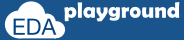# Implication Operator

```sequence seq;
@(posedge clk) a ##2 b;
endsequence
```

In the above sequence, we can observe that sequence starts on every positive edge of the clock and it looks for "a" to be high on every positive clock edge. If the signal "a" is not high on any given positive clock edge, an error is issued by the checker.

If we want the sequence to be checked only after “a” is high, this can be achieved by using the implication operator.

The implication is equivalent to an if-then structure. The left-hand side of the implication is called the "antecedent" and the right-hand side is called the "consequent." The antecedent is the gating condition. If the antecedent succeeds, then the consequent is evaluated.

The implication construct can be used only with property definitions. It cannot be used in sequences.

There are 2 types of implication:
• Overlapped implication
• Non-overlapped implication

## Overlapped implication

The overlapped implication is denoted by the symbol |->.

If there is a match on the antecedent, then the consequent expression is evaluated in the same clock cycle.

Below property checks that, if signal "a" is high on a given positive clock edge, then signal "b" should also be high on the same clock edge.

```property p;
@(posedge clk) a |-> b;
endproperty
a: assert property(p);
```

Execute the above code on## Non-overlapped implication

The non-overlapped implication is denoted by the symbol |=>.

If there is a match on the antecedent, then the consequent expression is evaluated in the next clock cycle.

Below property checks that, if signal "a" is high on a given positive clock edge, then signal "b" should be high on the next clock edge.

```property p;
@(posedge clk) a |=> b;
endproperty
a: assert property(p);
```

Execute the above code on### The implication with a fixed delay on the consequent

Below property checks that, if signal "a" is high on a given positive clock edge, then signal "b" should be high after 2 clock cycles.

```property p;
@(posedge clk) a |-> ##2 b;
endproperty
a: assert property(p);
```

Execute the above code on### The implication with a sequence as an antecedent

Below property checks that, if the sequence seq_1 is true on a given positive edge of the clock, then start checking the seq_2 (“d” should be low, 2 clock cycles after seq_1 is true).

```sequence seq_1;
(a && b) ##1 c;
endsequence

sequence seq_2;
##2 !d;
endsequence

property p;
@(posedge clk) seq_1 |-> seq_2;
endpeoperty
a: assert property(p);
```

Execute the above code on### Timing windows in SVA Checkers

Below property checks that, if signal "a" is high on a given positive clock edge, then within 1 to 4 clock cycles, the signal "b" should be high.

```property p;
@(posedge clk) a |-> ##[1:4] b;
endproperty
a: assert property(p);
```

Execute the above code on### Overlapping timing window

Below property checks that, if signal "a" is high on a given positive clock edge, then signal "b" should be high in the same clock cycle or within 4 clock cycles.

```property p;
@(posedge clk) a |-> ##[0:4] b;
endproperty
a: assert property(p);
```

Execute the above code on### Indefinite timing window

The upper limit of the timing window specified in the right-hand side can be defined with a "\$" sign which implies that there is no upper bound for timing. This is called the "eventuality" operator. The checker will keep checking for a match until the end of the simulation.

Below property checks that, if signal "a" is high on a given positive clock edge, then signal "b" will be high eventually starting from the next clock cycle.

```property p;
@(posedge clk) a |-> ##[1:\$] b;
endproperty
a: assert property(p);
```

Execute the above code on